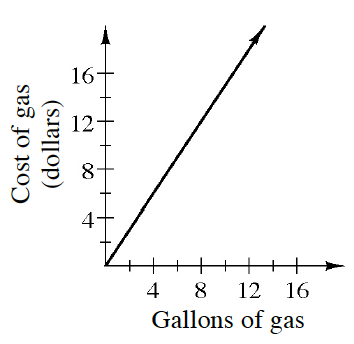Home > CCAA8 > Chapter cca1 > Lesson cca1.1.2 > Problem1-18

1-18.In December of 2003, the average price for a gallon of regular gas in the United States was $\1.50$. Homework Help ✎

1. At that time, what did it cost to buy $12$ gallons of gas?

Multiply the cost by the number of gallons to determine what the cost would be.

2. Gerald paid $\12.60$ for a tank of gas. How many gallons did he buy?

This is the opposite of part (a). You know what the cost is, so divide the final price by the cost per gallon.

3. At right is a graph of this situation. Predict how the line would change to represent the average cost of gas in December of 2005, when gas cost $\2.20$ per gallon on average.

The line would get steeper.